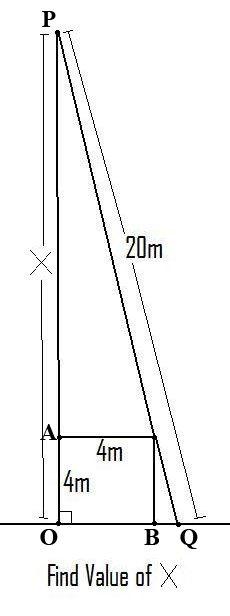Jim 10-12 other In case the pic doesn't work: Right angle triangle with a hypotenuse of 20 units. Square inside the triangle with sides of 4 units, the square shares two sides with both legs of the triangle, and the corner touches the hypotenuse limiting the triangles size. Hi Jim. I labeled some points on your diagram.Unfortunately I don't have a nice solution to your problem. Here is one solution. Let y = |PA| and x = |BQ| then using similar triangles y/4 = 4/x or x = 16/y Also triangle POQ is a right triangle and hence Pythagoras Theorem gives (y + 4)2 + (x + 4)2 = 202 Substitute x = 16/y and simplify. I got y4 + 8 y3 - 368 y2 + 128y + 256 = 0 I then used Mathematica to solve the quartic equation and got four solutions which numerically are y = 1.04, y = 15.35, y = -23.72 and y = -0.67 From the diagram the result you are looking for is y = 15.34 which symbolically is y = 2(-1 + Sqrt + Sqrt[23 - 2 Sqrt]) where Sqrt is the square root. Thus the length you want is 4 + 2(-1 + Sqrt + Sqrt[23 - 2 Sqrt]) which is approximately 19.35 meters. Penny